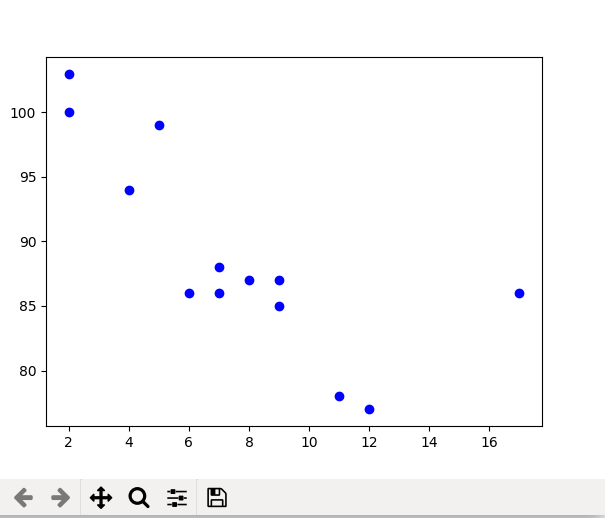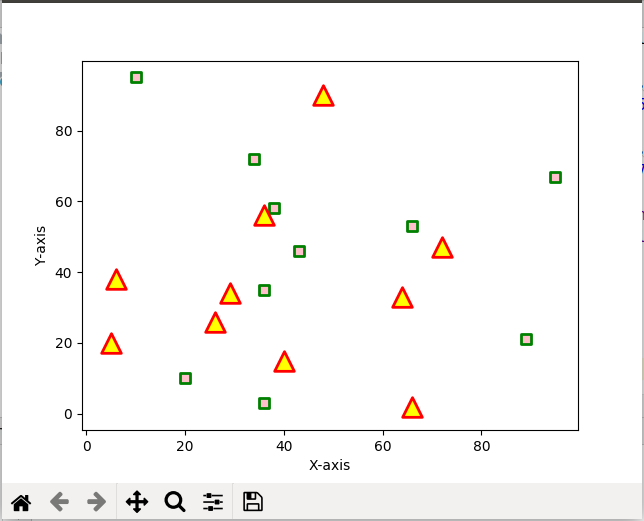# matplotlib.pyplot.scatter() in Python

## matplotlib.pyplot.scatter() in Python

Matplotlib is a comprehensive library for creating static, animated, and interactive visualizations in Python. It is used for plotting various plots in Python like scatter plot, bar charts, pie charts, line plots, histograms, 3-D plots and many more. We will learn about the scatter plot from the matplotlib library.

## matplotlib.pyplot.scatter()

Scatter plots are used to observe relationship between variables and uses dots to represent the relationship between them. The scatter() method in the matplotlib library is used to draw a scatter plot. Scatter plots are widely used to represent relation among variables and how change in one affects the other.
Syntax
The syntax for scatter() method is given below:

matplotlib.pyplot.scatter(x_axis_data, y_axis_data, s=None, c=None, marker=None, cmap=None, vmin=None, vmax=None, alpha=None, linewidths=None, edgecolors=None)

The scatter() method takes in the following parameters:

• x_axis_data- An array containing x-axis data
• y_axis_data- An array containing y-axis data
• s- marker size (can be scalar or array of size equal to size of x or y)
• c- color of sequence of colors for markers
• marker- marker style
• cmap- cmap name
• linewidths- width of marker border
• edgecolor- marker border color
• alpha- blending value, between 0 (transparent) and 1 (opaque)

Except x_axis_data and y_axis_data all other parameters are optional and their default value is None. Below are the scatter plot examples with various parameters.

Example 1: This is the most basic example of a scatter plot.

 `import` `matplotlib.pyplot as plt` ` ` ` ` `x ``=``[``5``, ``7``, ``8``, ``7``, ``2``, ``17``, ``2``, ``9``,` `    ``4``, ``11``, ``12``, ``9``, ``6``] ` ` ` `y ``=``[``99``, ``86``, ``87``, ``88``, ``100``, ``86``, ` `    ``103``, ``87``, ``94``, ``78``, ``77``, ``85``, ``86``]` ` ` `plt.scatter(x, y, c ``=``"blue"``)` ` ` `# To show the plot` `plt.show()`

OutputExample 2: Scatter plot with different shape and colour for two datasets.

 `import` `matplotlib.pyplot as plt` ` ` `# dataset-1` `x1 ``=` `[``89``, ``43``, ``36``, ``36``, ``95``, ``10``, ` `      ``66``, ``34``, ``38``, ``20``]` ` ` `y1 ``=` `[``21``, ``46``, ``3``, ``35``, ``67``, ``95``, ` `      ``53``, ``72``, ``58``, ``10``]` ` ` `# dataset2` `x2 ``=` `[``26``, ``29``, ``48``, ``64``, ``6``, ``5``,` `      ``36``, ``66``, ``72``, ``40``]` ` ` `y2 ``=` `[``26``, ``34``, ``90``, ``33``, ``38``, ` `      ``20``, ``56``, ``2``, ``47``, ``15``]` ` ` `plt.scatter(x1, y1, c ``=``"pink"``, ` `            ``linewidths ``=` `2``, ` `            ``marker ``=``"s"``, ` `            ``edgecolor ``=``"green"``, ` `            ``s ``=` `50``)` ` ` `plt.scatter(x2, y2, c ``=``"yellow"``,` `            ``linewidths ``=` `2``,` `            ``marker ``=``"^"``, ` `            ``edgecolor ``=``"red"``, ` `            ``s ``=` `200``)` ` ` `plt.xlabel(``"X-axis"``)` `plt.ylabel(``"Y-axis"``)` `plt.show()`

OutputLast Updated on March 1, 2022 by admin

## Pandas Series.to_frame()Pandas Series.to_frame()

Python | Pandas Series.to_frame() Pandas series is a One-dimensional ndarray with axis labels. The labels

## exec() in Pythonexec() in Python

exec() in Python exec() function is used for the dynamic execution of Python program which can

## Django ORM – Inserting, Updating & Deleting DataDjango ORM – Inserting, Updating & Deleting Data

Django ORM – Inserting, Updating & Deleting Data Django lets us interact with its database

## numpy.cov() functionnumpy.cov() function

Python | numpy.cov() function Covariance provides the a measure of strength of correlation between two

## Create a Snake-Game using Turtle in PythonCreate a Snake-Game using Turtle in Python

Create a Snake-Game using Turtle in Python A snake game is an arcade maze game which has

## callable() in Pythoncallable() in Python

callable() in Python In general, a callable is something that can be called. This built-in